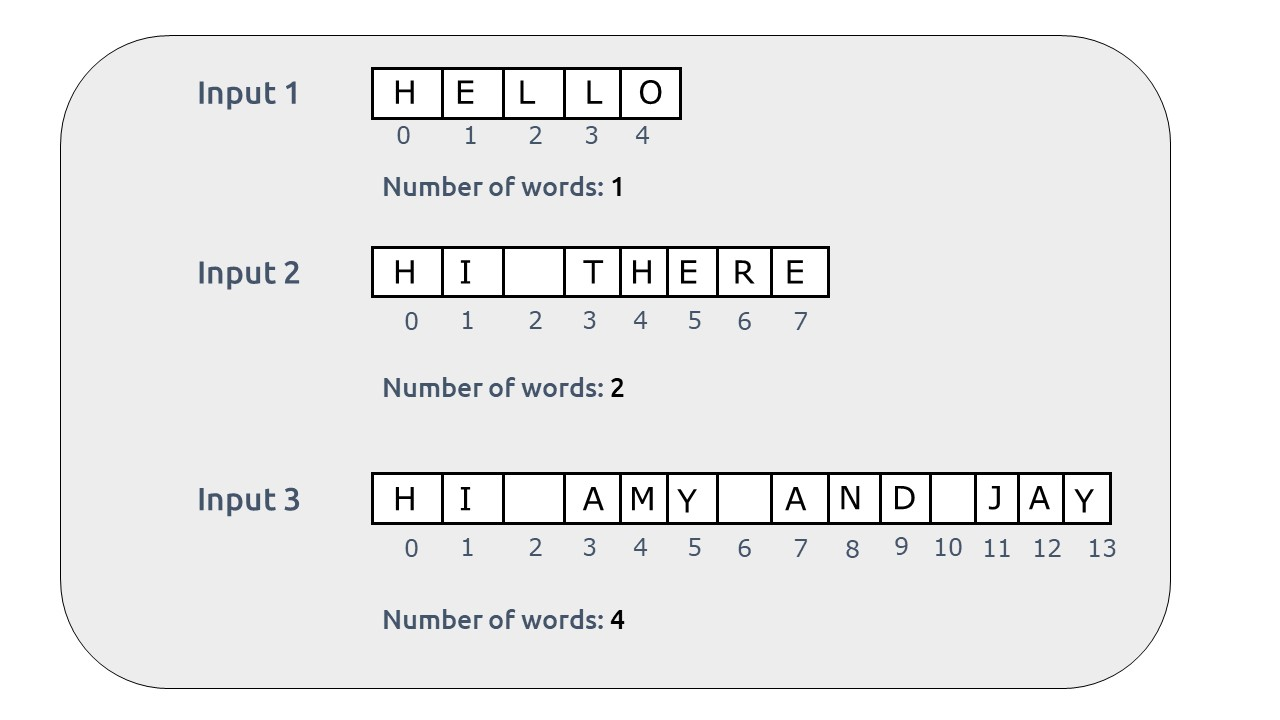# Count the number of words in a given string

Problem Statement: Write a program to count the number of words in a given string.

Examples:

``

Disclaimer: Don’t jump directly to the solution, try it out yourself first.

### Solution:

Intuition:

A string is a stream of characters. A word in a string is a bunch of characters surrounded by spaces on both sides or space on one side and string start/end on the other. In case a string is a single word, there will be no space around the word.Now, we can see by the observation that the number of words will always be (number of spaces + 1). To understand this, think of a log of wood. To get three pieces out of a single log, we need to make two cuts to the log. Here spaces are just like cuts, if there are 2 cuts, we get 3 pieces, similarly, if we have 2 spaces we have 3 words. Hence for ‘n’ spaces, the number of words will be ‘n+1’.Now we apply this formula to get the answer.Approach:

The algorithm steps are stated below:

• Iterate over the string and count the number of spaces and store it in a variable ( say spaces).
• Return the answer as (spaces + 1)

Code:

## C++ Code

``````#include <bits/stdc++.h>

using namespace std;

int main() {

string str = "HI AMY AND JAY";
int n = str.length();
int spaces=0;

for(int i=0; i<n; i++){
if(str[i]==' ')
spaces= spaces+1;
}

cout<<"The number of words are "<< spaces+1;

}
``````

Output: The number of words is 4

Time Complexity: O(N)

Reason: We iterate for the length of the string

Space Complexity: O(1)

Reason: We are not using any extra space.

## Java Code

``````import java.io.*;
import java.util.*;

class takeuforward {

// Driver code
public static void main(String[] args) {
String str = "HI AMY AND JAY";
int n = str.length();
int spaces = 0;

for (int i = 0; i < n; i++) {
if (str.charAt(i) == ' ')
spaces = spaces + 1;
}

System.out.print("Number of words are ");
System.out.print(spaces + 1);
}
}
``````

Output: The number of words is 4

Time Complexity: O(N)

Reason: We iterate for the length of the string

Space Complexity: O(1)

Reason: We are not using any extra space.